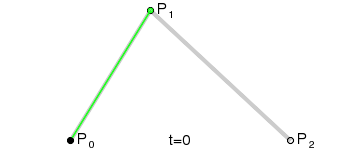# How to tween/lerp a Bezier curve

As seen from a few posts that I find, the result I can get is either keep on stopping and continuing the tween, or use lerp which continuously changes the CFrame, which I don’t know, is how do you actually get the “Position” or CFrame of the certain point in the curve, as you need that point to tween/lerp to. From the Dev Hub i can find the formula, but the formula only returns a position value, which I don’t think can be used to do the curve, it will be appreciated to have a solution to this.Thanks.

2 Likes

Bezier functions usually take in a number from 0-1 that represents a percent along the curve:

``````local function QuadraticBezier(t, p0, p1, p2)
local a = p0:Lerp(p1, t)
local b = p1:Lerp(p2, t)
return a:Lerp(b, t)
end
``````If you wanted to get the point at the start of that curve, you’d provide a `t` of 0.

If you wanted to get the point in the middle of that curve, you’d provide a `t` of 0.5, etc.

4 Likes

sorry about my explanation, I know how the curve works, but what I dont know is how to make a tween/lerp thats moves along the curve. Thats why i want to find a certain “position” on the curve because i need to tween it to. Thanks

I’m still a bit confused sorry. What are your inputs and what output do you want?

Maybe it would help if you said what you’re using this for at a higher level?

I am basically trying to make a curve between 2 points(the bezier curve), and make an object move along that curve, using the gif you put above, i am trying to make the black dot that is moving along the curve. Sorry about my bad english i don’t have it as my first language. Thanks for helping.Oh! All you have to do is move some value from 0 to 1 and compute the position.

I talk about it in this post:

(In that I call `QuadraticBezier`, which was defined earlier in that thread, to compute the position but you can substitute your own bezier function)

I can understand what you mean by the post there, but the reason i am using this is to make a curved path for a vehicle to drive along, and because I use tween for straight lines, is there a way to keep on updating the tween in order to move along the curve? Or should i just stick to the one you mentioned above, Thanks for the help.

Can you maybe share the code you’re using for straight paths, and we could translate it to use bezier?

I actually attempted this a while back and can share my code hold on 1 second

Ok here you go I made it into a model this version is actually cubic bezier which requires 4 points but you can easily change it to 3.

https://www.roblox.com/library/6696416877/Cubic-Bezier-model

3 Likes

I will take a look at that later, thanks for the helpIts just a basic tween to CFrame with {CFrame=part.CFrame} whereas part is the part to move to

1 Like

You could change it to tween but as it’s in a loop it already looks smooth enough it depends on how fast you want the part to move.

I have just taken a look at the model, and i kinda get what i needed. Thanks for you help, appreciated.

1 Like